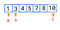# Count Triplets — Day 28(Python)Triplets(Pinterest)

Today I will be solving a question that I encountered during an online assessment for a FinTech company. I found it quite interesting and thought it would be great to share it on Medium. Let’s jump into the problem without wasting much time.

# Count triplets with a sum smaller than a given value.

This question is from GeeksForGeeks.

Given an array of distinct integers and a sum value. Find the count of triplets with a sum smaller than the given sum value. The Expected Time Complexity is O(n²).

Examples:

`Input : arr[] = {-2, 0, 1, 3}        sum = 2.Output : 2Explanation :  Below are triplets with sum less than 2               (-2, 0, 1) and (-2, 0, 3) Input : arr[] = {5, 1, 3, 4, 7}        sum = 12.Output : 4Explanation :  Below are triplets with sum less than 12               (1, 3, 4), (1, 3, 5), (1, 3, 7) and                (1, 4, 5)`

One of the easiest ways to solve this problem is by using 3 for loops.

Have a counter initialized as 0.

The first loop will run from the beginning until last but two and get the first number.

The second loop will run from the number after the first number until the last but one and get the second number.

The third loop will run from the number after the second number until the last and get the third number.

Get all three numbers and find the sum for these three numbers. If the sum is less than the given value, increase the value of the counter.

Let us look at how to code it.

`class TripletCounter:    def countTriplets(self, arr, n, sum):        counter = 0        for a in range(len(arr)-2):            for b in range(a+1, len(arr)-1):                for c in range(b+1, len(arr)):                    if arr[a] + arr[b] + arr[c] < sum:                        counter += 1        return counter`

Complexity analysis.

Time Complexity

We are using three loops while using the above code. Hence the time complexity is O(N³).

Space Complexity

We are not using any extra data structure to store any intermediate results, and hence the space complexity is O(1).

Can we try to optimize the solution? What if we try to sort the array?

Let us take an example and solve it. We have the following array and sum value.

`arr = [5, 1, 3, 4, 7, 8, 10]`

`sum` `=` `12`

Let’s sort the given array → `arr = [1, 3, 4, 5, 7, 8, 10]`

Consider “a” as index 0 and “b” as index 1, and “c” as index 6.Initializing the variables

The sum of the given variables is 14, which is greater than the required sum. Hence we shift c to the left by 1.

The sum of the given variables is 12, which is greater than the required sum. Hence we shift c to the left by 1.

The sum of the given variables is 11, which is lesser than the required sum. Do we need to move c any further? Since we have sorted the array, we just need to find the number of elements between b and c and add it to the counter. Continue the above steps until “a” reaches last but two.

`class TripletCounter:    def countTriplets(self, arr, n, sum):        arr.sort()        counter = 0        for i in range(0,n-2):            j = i + 1            k = n-1            while(j < k):                 if (arr[i]+arr[j]+arr[k] >=sum):                        k = k-1                 else:                        ans += (k - j)                        j = j+1        return ans`

Complexity analysis.

Time Complexity

We are using 2 loops while using the above code. Hence the time complexity is O(N²).

Space Complexity

We are not using any extra data structure to store any intermediate results, therefore the space complexity is O(1).

I would love to hear your feedback about my posts. Do let me know if you have any comments or feedback.

## More from Annamariya Tharayil

Software Engineer. Find me @ www.linkedin.com/in/annamariya-jt